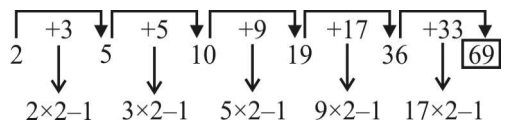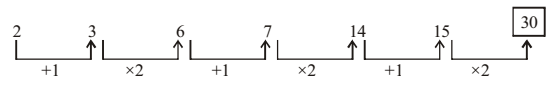## Number Series

#### Number Series

Direction: In each of the following questions, a number series is given in which one number is wrong. You have to find out that number and have to follow the new series which will be started by that number. By following this, which will be the second number of the new series?

1. 7, 23, 58, 127, 269, 555
1. 263
2. 261
3. 299
4. 286
5. None of these

1. The order of above series is given as
First term = 7
Second term = First term x 2 +9
Third term = Second term x 2 +11
Similarly we can calculate next terms also.

##### Correct Option: B

The order of above series is given as
First term = 7
Second term = ( First term x 2 ) +9
Second term = ( 7 x 2 ) + 9 = 14 + 9 = 23
Third term = ( Second term x 2 ) +11
Third term = ( 23 x 2 ) + 11 = 46 + 11 = 58 is wrong , 57 should be Third term because ( 23 × 2 ) + 11 = 57 .
Similarly we can find all others terms also.
Fourth term = ( Third term x 2 ) +13
Fourth term = ( 57 x 2 ) +13
Fifth term = ( Fourth term x 2 ) +15
Fifth term = ( 127 × 2 ) + 15 = 269
Sixth term = ( Fifth term x 2 ) +17
Sixth term = ( 269 × 2 ) + 17 = 555

Direction: What will come in the place of question mark (?) in the following number.

1. 156, 506, ?, 1806
1. 1056
2. 856
3. 1456
4. 1506
5. None of these

1. From the above given series , we have
First term + 350 = Second term
⇒ 156 + 350 = 506
Second term + 550 = third term
⇒ 506 + 550 = ? ⇒ ? = 1056
..................................
...................................

##### Correct Option: A

From the above given series , we have
First term + 350 = Second term
⇒ 156 + 350 = 506
Second term + 550 = third term
⇒ 506 + 550 = ? ⇒ ? = 1056
From the given figure , we get the value of third term ( ? ) = 1056
Third term + 550 = Fourth term
⇒ 1056 + 750 = 1806

1. 2, 5, 10, 19, 36, ?
1. 70
2. 71
3. 68
4. 69
5. None of these

1. See the below image and find the pattern to solve this question.

##### Correct Option: D

See the below image and you will find a pattern in which numbers are getting added for next number in the given series.
From the given figure , we get the value of sixth term ( ? ) = 691. 2, 3, 6, 7, 14, 15, ?
1. 16
2. 30
3. 31
4. 32
5. None of these

1. See the graph and found that the given number in the series with specific pattern.
From the given graph , we can see that the increasing order of series as -
First term = 2
Second term = First term + 1 = 3 ,
Third term = Second term x 2 = 6
Similarly , we can calculate all others terms also.

##### Correct Option: B

See the graph and found that the given number in the series with specific pattern.
From the given graph , we can see that the increasing order of series as -
First term = 2
Second term = First term + 1 = 2 + 1 = 3 ,
Third term = Second term x 2 = 3 x 2 = 6 ,
Fourth term = Third term + 1 = 6 + 1 = 7 ,
Fifth term = Fourth term x 2 = 7 x 2 = 14 ,
Sixth term = Fifth term + 1 = 14 + 1 = 15 ,
Seventh term = Sixth term x 2 = 15 x 2 = 30
Seventh term( ? ) = 301. BCFG, JKNO, RSVW , ?
2. HIKL
3. STUX
4. MNPQ
5. None of these

1. According to position of alphabets in alphabet table , we have
B C F G → 2 , 3 ,6 , 7
J K N O → 10 , 11 , 14 , 15
R S V W → 18 , 19 , 22 , 23
{ ∴ Next sequence = Adding 8 in every position of previous sequence alphabets }

##### Correct Option: A

According to position of alphabets in alphabet table , we have
B C F G → 2 , 3 ,6 , 7
J K N O → 10 , 11 , 14 , 15
R S V W → 18 , 19 , 22 , 23
{ ∴ Next sequence = Adding 8 in every position of previous sequence alphabets }
Next sequence = 18 + 8 , 19 + 8 , 22 + 8 , 23 + 8
Next sequence = 26, 27, 30, 31 = 26, 27 - 26 = 1, 30 - 26 = 4, 31 - 26 = 5 = Z A D E ( subtract the excess value by 26 )
We get , 26 , 1 , 4 , 5Z A D E = ( ? ) .